Fall 2003

# Mathematics Math21b Fall 2003

## Linear Algebra and Differential Equations

Office: SciCtr 434
Email: knill@math.harvard.edu# PDE's (Solution by diagonalization)

Some PDE's like the heat or wave equation
 ``` ft(t,x) = fxx(t,x) ftt(t,x) = fxx(t,x) ```
can be solved similarly as ODE's.
 ``` xt (t) = A x(t) xtt(t) = A x(t) ```
Diagonalizing A
 ``` A v=L v A v=-n2 v A v=0 ```
on eigenspaces led to
 ``` v'=L v v''=-n2 v v''=0 ```
which are solved by
 ``` v(t)=exp(Lt) v v(t)=v(0) cos(nt)+v'(0) sin(nt)/n v(t)=v(0)+t v'(0) ```
Diagonalizing T=D2
 ```T sin(nx)=-n2sin(nx), T cos(nx)=-n2cos(nx), T 21/2 = 0. ```
 ```f'(t)=-n2f(t) f''(t)=-n2f(t) f''(t)=0 ```
which are solved by
 ```f(t)=exp(-n2 t) f(0) f(t)=cos(nt) f(0)+sin(nt)/n f'(0) f(t)=f(0)+t f'(0) ```
For f written in the Fourier basis
 f(0,x) = a0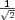+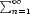an cos(n x) + bn sin(n x)
we obtain a solution to the heat equation
 f(t,x) = a0+an exp(-n2 t) cos(n x) + bn exp(-n2 t) sin(n x)
For f,f' written in the Fourier basis as
 f(0,x) = a0+an cos(nx) + bn sin(nx) f' (0,x) = a'0+a'n cos(nx) + b'n sin(nx)
we obtain a solution to the wave equation
 f(t,x) = (a0+a'0 t)+(an cos(nt)+a'n sin(nt)/n) cos(nx) +(bn cos(nt) + b'n sin(nt)/n) sin(nx)

## Fourier decomposition

 In this particular case, the Fourier coefficients bn belonging to sin(nx) are zero because the function is an even function. Also an is zero here if n is even.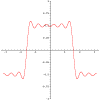=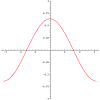+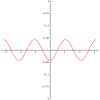+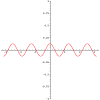+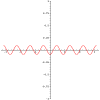++ f(x) = a1 cos(x) + a3 cos(3x) + a5 cos(5x) + a7 cos(7x) + a9 cos(9x) +

## Heat evolution

 The heat evolution is simple on each eigenfunction f=cos(nx) of D2. Since f' = -n2 f for such functions, the decay is fast, if n is large.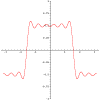=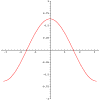+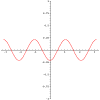+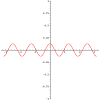+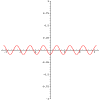++

## Wave evolution

 The wave evolution is simple on each eigenfunction f=cos(nx) of D2. Since f'' = -n2 f for such functions, the waves oscillate fast for small wavelength.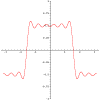=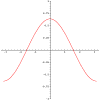+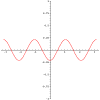+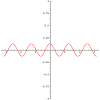+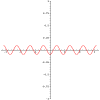++

## Using Symmetry

 Remarks. In the case, when we are interested in the evolution on an interval like [0,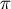], one can flip the graph at the y axes to obtain an even function f(x)=f(-x) on [-,] which has a pure cos series. For functions which are zero at 0 and, it makes also sense to continue on the left to an odd function f(x)=-f(x) which has a sin Fourier series. This simplifies the formulas.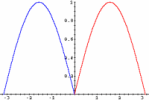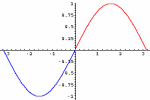## 2D heat and wave equation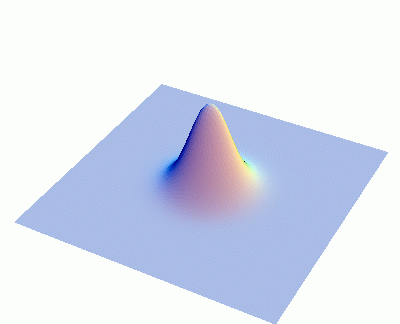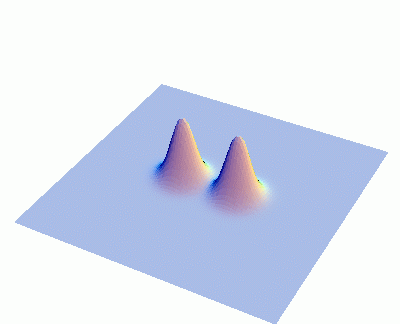Back to the main page